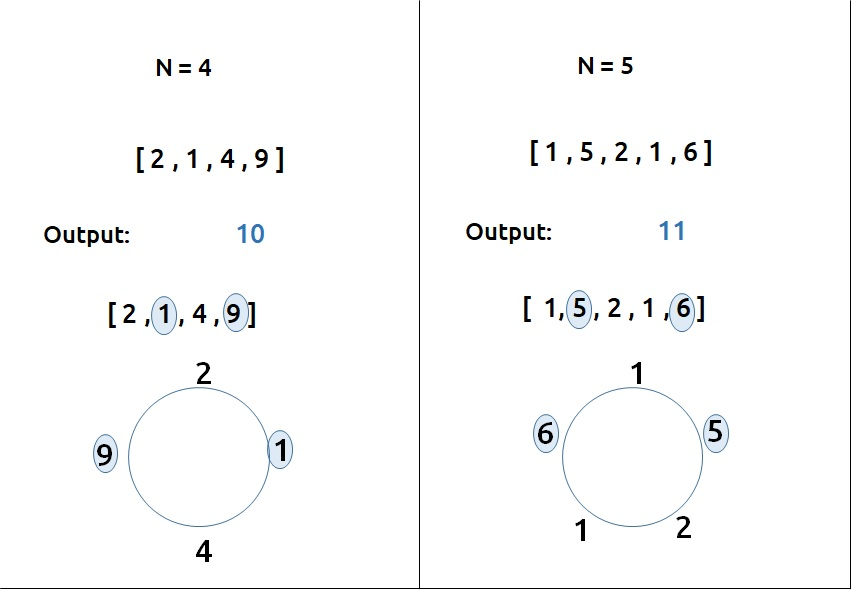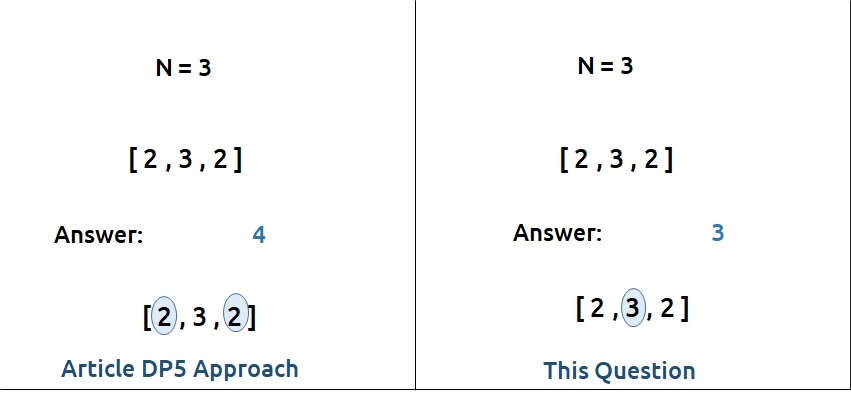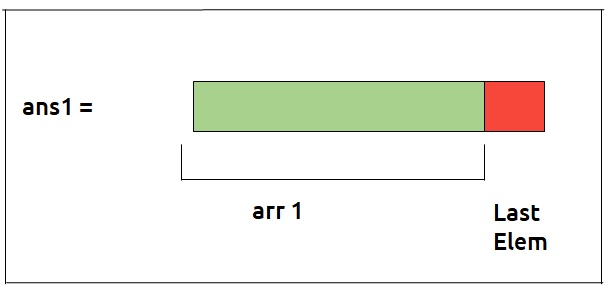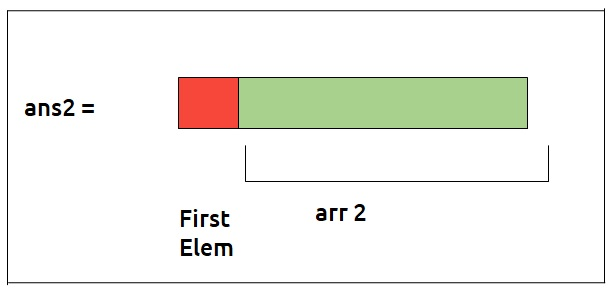# Dynamic Programming: House Robber (DP 6)

Problem Statement: Dynamic Programming: House Robber (DP 6)

Problem Statement Link: House Robber

A thief needs to rob money in a street. The houses in the street are arranged in a circular manner. Therefore the first and the last house are adjacent to each other. The security system in the street is such that if adjacent houses are robbed, the police will get notified.

Given an array of integers “Arr” which represents money at each house, we need to return the maximum amount of money that the thief can rob without alerting the police.

```Examples:```

### Solution :

This question can be solved using the approach discussed in the Maximum Sum of non-adjacent elements. Readers are highly advised to go through that article first and then read this. The rest of the article will refer to the previous article as Article DP5 and will relate to that approach.

Now, we have a single test case. Three houses have money as shown.• According to article DP5, the answer will be 4(2+2) as we are taking the maximum sum of non-adjacent elements.
• In this question, the first and last element are also adjacent(circular street), therefore the answer will be 3.

Modification to Article DP5’s Approach

We were finding the maximum sum of non-adjacent elements in the previous questions. For a circular street, the first and last house are adjacent, therefore one thing we know for sure is that the answer will not consider the first and last element simultaneously (as they are adjacent).

Now building on the article DP5, we can say that maybe the last element is not considered in the answer. In that case, we can consider the first element. Let’s call this answer ans1. Hence we have reduced our array(arr- last element), say arr1, and found ans1 on it by using the article DP5 approach.Now, it can also happen that the final answer does consider the last element. If we consider the last element, we can’t consider the first element( again adjacent elements). We again use the same approach on our reduced array( arr – first element), say arr2. Let’s call the answer we get as ans2.

Now, the final answer can be either ans1 or ans2. As we have to return the maximum money robbed by the robber, we will return max(ans1, ans2) as our final answer.Approach:

The approach to solving this problem can be summarized as:

• Make two reduced arrays – arr1(arr-last element) and arr2(arr-first element).
• Find the maximum of non-adjacent elements as mentioned in article DP5 on arr1 and arr2 separately. Let’s call the answers we got as ans1 and ans2 respectively.
• Return max(ans1, ans2) as our final answer.

Code:

``````#include <bits/stdc++.h>

using namespace std;

long long int solve(vector<int>& arr){
int n = arr.size();
long long int prev = arr;
long long int prev2 =0;

for(int i=1; i<n; i++){
long long int pick = arr[i];
if(i>1)
pick += prev2;
int long long nonPick = 0 + prev;

long long int cur_i = max(pick, nonPick);
prev2 = prev;
prev= cur_i;

}
return prev;
}

long long int robStreet(int n, vector<int> &arr){
vector<int> arr1;
vector<int> arr2;

if(n==1)
return arr;

for(int i=0; i<n; i++){

if(i!=0) arr1.push_back(arr[i]);
if(i!=n-1) arr2.push_back(arr[i]);
}

long long int ans1 = solve(arr1);
long long int ans2 = solve(arr2);

return max(ans1,ans2);
}

int main() {

vector<int> arr{1,5,1,2,6};
int n=arr.size();
cout<<robStreet(n,arr);
}
``````

Output: 11

Time Complexity: O(N )

Reason: We are running a simple iterative loop, two times. Therefore total time complexity will be O(N) + O(N) ≈ O(N)

Space Complexity: O(1)

Reason: We are not using extra space.

## Java Code

``````import java.util.*;
class TUF{
static long solve(ArrayList<Integer> arr){
int n = arr.size();
long prev = arr.get(0);
long prev2 =0;

for(int i=1; i<n; i++){
long pick = arr.get(i);
if(i>1)
pick += prev2;
long nonPick = 0 + prev;

long cur_i = Math.max(pick, nonPick);
prev2 = prev;
prev= cur_i;

}
return prev;
}

static long robStreet(int n, ArrayList<Integer> arr){
ArrayList<Integer> arr1=new ArrayList<>();
ArrayList<Integer> arr2=new ArrayList<>();

if(n==1)
return arr.get(0);

for(int i=0; i<n; i++){

}

long ans1 = solve(arr1);
long ans2 = solve(arr2);

return Math.max(ans1,ans2);
}

public static void main(String args[]) {

ArrayList<Integer> arr=new ArrayList<>();
int n=arr.size();
System.out.println(robStreet(n,arr));

}
}``````

Output: 11

Time Complexity: O(N )

Reason: We are running a simple iterative loop, two times. Therefore total time complexity will be O(N) + O(N) ≈ O(N)

Space Complexity: O(1)

Reason: We are not using extra space.

Special thanks to Anshuman Sharma for contributing to this article on takeUforward. If you also wish to share your knowledge with the takeUforward fam, please check out this article